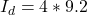## A parallel-plate capacitor with circular plates of radius R is being discharged. The displacement current through a central circular area, p

Question

A parallel-plate capacitor with circular plates of radius R is being discharged. The displacement current through a central circular area, parallel to the plates and with radius R/2, is 9.2 A. What is the discharging current?

in progress 0
3 months 2021-08-17T01:51:23+00:00 1 Answers 6 views 0

The discharging current is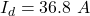Explanation:

From the question we are told that

The radius of each circular plates is  R

The displacement current is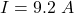The radius of the central circular area is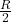The discharging current is mathematically represented as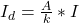where A is the area of each plate which is mathematically represented as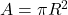and   k is central circular area which is mathematically represented as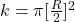So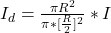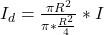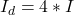substituting values